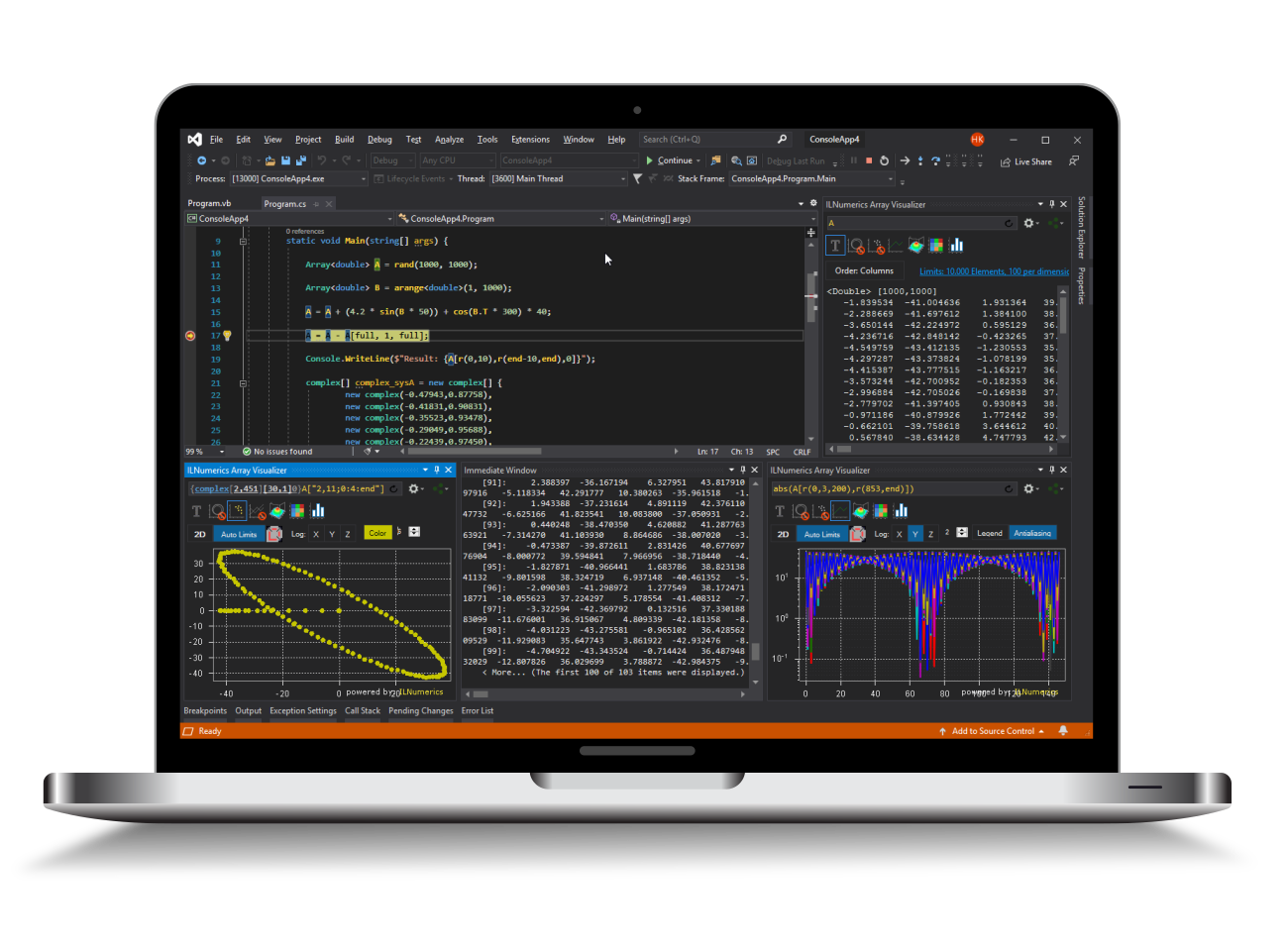Industrial Data Science
in C# and .NET:
Simple. Fast. Reliable.ILNumerics - Technical Computing

Modern High Performance Tools for Technical

Computing and Visualization in Industry and Science

# Stacked area plot

StackedAreaPlot enables you to create stacked area plots and layered area plots. This functionality is available since version 4.13 in the ILNumerics Drawing Extensions Toolbox.

### Using StackedAreaPlot

To create an StackedAreaPlot object, the following input parameters must be provided:

• A [1 x n] vector X containing the horizontal positions of the stacked area plot respectively layered area plot
• A [m x n] matrix Y containing the vertical spacing  respectively vertical positions

The graph below shows a stacked area plot:

Once an StackedAreaPlot object exists, the method Update() can be used to modify the input parameters X, Y and spacing. Note, that all input parameters are optional. spacing will only have an effect on layered area plots, which is described in detail below. The following example displays a case of application:

Be aware, that the input parameters must match in terms of size. So let's say you decide to only change the input parameter X, its numbers of columns must still match the number of columns of the input parameter Y that was provided before.

### Layered Area Plot

As mentioned above, StackedAreaPlot also enables you to create layered area plots as shown in the example below:

The input parameter spacing determines the distance between the separate area plots on the Z-axis. If you prefer using layered area plots, you only have to set the input parameter isLayeredAreaPlot to true.

### Optional parameters

Next to the mandatory input parameters, there is also a set of optional parameters such as:

• isLayeredAreaPlot determines whether a stacked area plot or a layered area plot is created
• spacing specifies the distance of the area plots on the Z-axis
• colormap determines the colors of the area plots
• fillColor specifies the color of the area of the area plots
• lineColor contains the color of  border of the area plots
• transparency indicates the alpha value for each area plot

ILNumerics offers a series of built-in colormaps but you may also specify your own, as explained in this tutorial. StackedAreaPlot maps the Z-values of the area plots, assigning a specific color to each area plot. However, you can also choose to use the same color for all area plots, by specifiying the input parameter fillColor. To be able to better distinguish the area plots, you might want to use it in combination with the input parameter transparency. Note that if you choose to specify the transparency, you must provide an alpha value for each area plot. Furthermore, you can color the borders of the area plots by determining the input parameter lineColor.

The following plot displays a customized layered area plot:

If you want to go into more detail, you can use the following statements to get access to a specific area plot:

First, the IDs of all area plots included in the StackedAreaPlot object from above are yielded. With the help of the ID a specific area plot is adressed. In this way all of its properties can be modified.

Of course, the same options are available for stacked area plots.

### Further configurations

Since StackedAreaPlot derives from Group, you have access to all configuration options of common group objects.